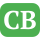CppBuzz.com

## Home » C++ » C++ 11 Multithreading Examples

C++ 11 has introduced its own thread class which has functions related to multithreading

This C++ code shows the basic program which create one thread and execute it

```#include <iostream>

{
}

int main()
{
std::cout << "this is main thread\n";
t.join();   // <- main thread waits for the thread t to finish

return 0;
}
```

This code will ouput (linux system):

We can make a new thread to run free to become a daemon process.

```int main()
{
// t.join();
t.detach();
return 0;
}
```

The detached child thread is now free, and runs on its own. It becomes a daemon process.

Note that the detached thread didn't have a change to print its output to stdout because the main thread already finished and exited. This is one of the characteristics of multithreaded programming: we cannot be sure which thread runs first (not deterministic unless we use synchronization mechanism). In our case, because the time it takes to create a new thread, the main thread is most likely to finish ahead of our child thread.
One more thing we should note here is that even in this simple code we're sharing a common resource: std::cout. So, to make the code work properly, the main thread should allow our child thread to access the resource.
Once a thread detached, we cannot force it to join with the main thread again. So, the following line of the code is an error and the program will crash.

```int main()
{
// t.join();
t.detach();
t.join();   // Error
return 0;
}
```

Once detached, the thread should live that way forever.
We can keep the code from crashing by checking using joinable(). Because it's not joinable, the join() function won't be called, and the program runs without crash.

```int main()
{
// t.join();
if(t.joinable())
t.join();
return 0;
}
```

## Passing Parameters to a thread

Here is an example of passing parameter to a thread. In this case, we're just passing a string:

```#include <iostream>
#include <string>

{
std::cout << "message is = " << s << std::endl;
}

int main()
{
std::string s = "CppBuzz";
std::cout << "main thread message = " << s << std::endl;
t.join();
return 0;
}
```

From the following output, we know the string has been passed to the thread function successfully.

thread function message is = CppBuzz

If we want to pass the string as a ref, we may want to try this:

```
{
std::cout << "message is = " << s << std::endl;
s = "Chicago";
}
```

To make it sure that the string is really passed by reference, we modified the message at the end of the thread function. However, the output hasn't been changed.

thread function message is = CppBuzz

In fact, the message was passed by value not by reference. To pass the message by reference, we should modify the code a little bit like this using ref:

```
```

Then, we get modified output:

thread function message is = CppBuzz

There is another way of passing the parameter without copying and not sharing memory between the threads. We can use move():

```
```

Since the string moved from main() to thread function, the output from main does not have it any more:

thread function message is = CppBuzz

```
#include <iostream>

{
}

int main()
{

t2.join();

return 0;
}
```

But we can transfer the ownership of the thread by moving it:

```
#include <iostream>

{
}

int main()
{

t2.join();

return 0;
}
```

Output:

We can get id information using this_thread::get_id():

```
int main()
{
std::string s = "CppBuzz";
std::cout << "main thread message = " << s << std::endl;

std::cout << "child thread id = " << t.get_id() << std::endl;

t.join();
return 0;
}
```

Output:

thread function message is = CppBuzz

```
int main()
{
std::cout << "Number of threads = "